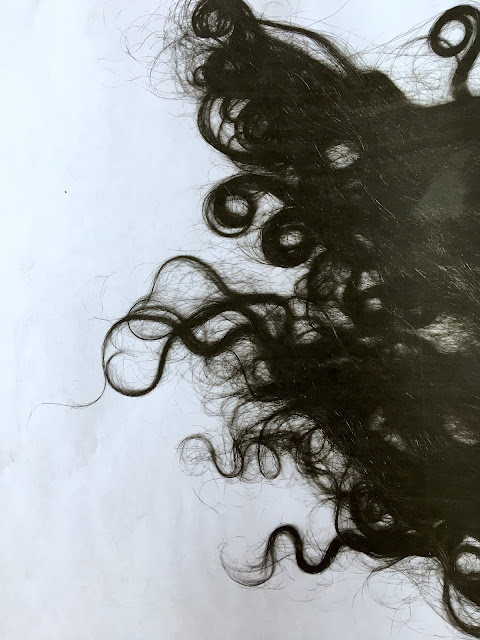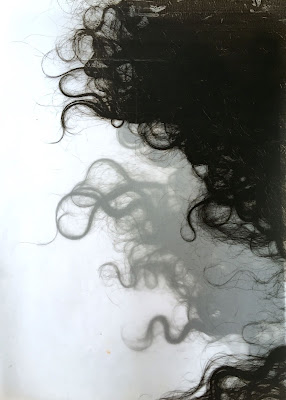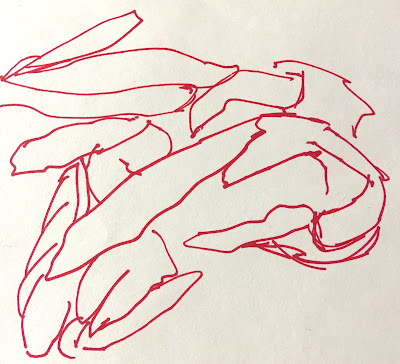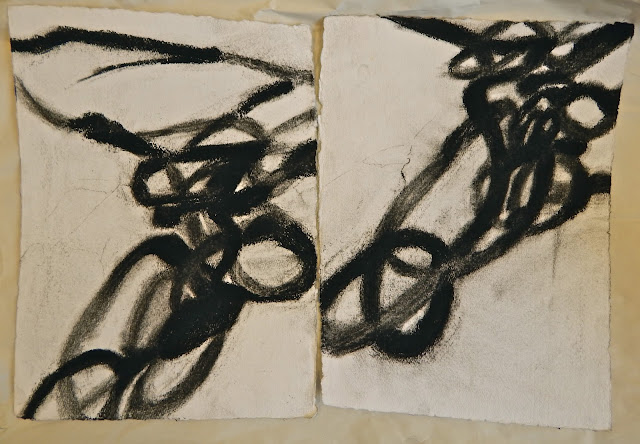## Pages

Showing posts with label metaphors. Show all posts
Showing posts with label metaphors. Show all posts

## May 2, 2019

### Making it up the mountain

I've been making my way up the mountain. That's both real and metaphorical.

The walk up Beacon mountain starts just three houses from mine. I've climbed to the top a bunch of times, but lately it seems like more of a struggle.

Today I'm happy to say I made it even further up.
The bugs getting stuck in my hair were actually more of a challenge than the steep climb. Which reminded me of course of a song my father used to sing to me. Because most things in life remind me of a song my father used to sing to me.

Hair from the play Hair:
"Darlin', give me a head with hair, long beautiful hair. Shining, gleaming, steaming, flaxen, waxen. Give me down to there hair, shoulder length or longer. Hair, hair, hair, hair, hair, hair, hair, hair. I want it long, straight, curly, fuzzy, snaggy, shaggy, ratty, Matty, oily, greasy, fleecy, shining, gleaming, streaming, flaxen, waxen. Hair, hair, hair, hair."

I have this idea to make a book of photocopies of my hair. Here are the first two.## March 19, 2019

### IBS: An Exhibition Proposal

I n s i d e   a n d   O u tSamantha Palmeri, untitled drawing

I   a m   s o   s l o w ,   i n s i d e   a n d   o u t .   I ' m   s l o w   t o   a b s o r b   a n d   r e c o g n i z e ,   s l o w   t o   p r o c e s s ,   a n d   s l o w   t o   a c c e p t .   I ' m   s o   s l o w   i t ' s   t a k e n   m e   4 5   y e a r s   t o   r e a l i z e   h o w   s l o w   I   a m .   I t ' s   t a k e n   m e   h a l f   a   l i f e t i m e   t o   c o n n e c t   t h e       d o t s   i n   m y   l i f e ,   s o m e   t h a t   a r e   p r o b a b l y   v e r y   o b v i o u s   t o   a n y o n e   l o o k i n g .   T h i n g s   t h a t   h a p p e n   i n   c h i  l d h o o d   o f t e n   b e c o m e   t h i n g s   t h a t   y o u   m a k e   h a p p e n   i n   a d u l t h o o d .   I r o n i c a l l y   I ' m   a   v e r y   i m p a t i e n t   p e r s o n   w h e n   i t     c o m e s   t o   m y   f e e l i n g s ,   a n d   t h i s   t o r t o i s e   l i k e   s l o w   p r o c e s s   d o e s   n o t  b o d e   w e l l   w i t h   m y   u r g e n c y .

E v e n   m y   b o d y   i s   s l o w .   I   i n t e r n a l i z e   e v e r y t h i n g .  I   d o n ' t   t h i n k   i t ' s   i r o n i c   t h a t   I   h a v e   d i g e s t i o n   p r o b l e m s .   Y o u   c a n   o n l y   s w a l l o w   d o w n   s o   m u c h   b e f o r e   t h i n g s   g e t   t e r r i b l y   b a c k e d   u p .  I ' m   l i k e   a   d u m p  t r u c k  t h a t  g e t s   f i l l e d   a n d   e m p t i e d ,   f i l l e d   a n d   e m p t i e d .

T a k e   t h e   d i g e s t i v e   s y s t e m .   Y o u   i n g e s t   f o o d ,   y o u r   b o d y   d i g e s t s   i t ,   a n d   i t   c o m e s   o u t   o f   y o u .  F o r   p e o p l e   w i t h   I B S   l i k e   m e ,  t h a t   s y s t e m   d o e s n ' t  w o r k   p r o p e r l y . I n   s o m e   c a s e s   y o u   i n g e s t   f o o d ,   y o u r   b o d y   d o e s n ' t   d i g e s t   i t ,   a n d   i t   s t a y s   f o r   t o o   l o n g   u n t i l   i t   f i n a l l y   e x p l o d e s   o u t   o f   y o u .     M y   h u s b a n d   h a s   t o l d   m e   t h i s   i s   a   l o t   l i k e   m y   p e r s o n a l i t y ,   t h a t  I  b o t t l e   t h i n g s   u p   u n t i l   I   e x p l o d e ,   u s u a l l y   w h e n   w e   l e a s t   e x p e c t   i t .

M y   p h y s i c a l   b o d y   s e e m s   t o   b e   m a n i f e s t i n g   t h e   i n v i s i b l e   f o r c e s   t h a t     m a k e   u p   m y   h e a r t   a n d   m i n d .

I ' v e   b e e n   t h i n k i n g   a b o u t   a l l   t h e s e   t h i n g s   l i k e   m a y b e   t h e r e ' s   a n   e x h i b i t i o n   h e r e .   M a y b e   t h i s   i s   a   g o o d   s t o r y   f o r   a   n e w   s e r i e s    o f   p a i n t i n g s .      T h e   s e r i e s   c o u l d   b e   a l l   v e r y   l a r g e   d i p t y c h s   t o   r e p r e s e n t   t h e   d u a l i t y     o f   v e r y   l a r g e   h a l f   d i g e s t e d   t r u t h s .   P e r h a p s   t h e r e   w o u l d   b e   l o t s   o f   i r o n y .   A n d   i t   w o u l d   b e  t i t l e d   I n s i d e   a n d   O u t .

## September 30, 2015

### unavoidable metaphors everywhere

here's what I just learned this week: that muscles do not actually grow bigger the more you work them out. muscles develop tiny little tears that repair themselves, which makes them thicker and stronger than they were before. the body repairs the damaged muscle with new and improved muscle protein. in other words, muscles need to break down before they can build themselves up. and interestingly, this does not happen while you're actually doing the workout, it happens while you rest, after the workout.

I think that is amazing. I think it's amazing that God would make it that way (yes... I said God), and it makes me wonder and imagine all the million billion minute details of our bodies and our lives that God has also made that way.

in essence, nothing was really meant to be easy. we have to just figure out which battles are the most important, and how to carve out little moments of peace for ourselves.2 drawings from 2 days ago

today I plan to carve out some more charcoal drawings for myself, a similar process of breaking down and building up. like both a battle and a moment of peace at the same time.